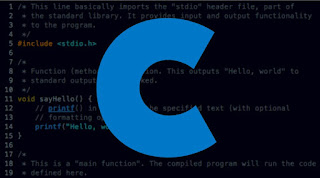Lompat ke konten Lompat ke sidebar Lompat ke footer

# How do I write a program to find greater among three numbers in C language?How do I write a program to find greater among three numbers in C language?

The code in C Language to calculate the greate of three numbers.

This is a common programming question which is asked at various levels to gather the thought process of the candidate or the person who is trying to solve the problem.

There are various ways we can solve this problem and we must take care of the boundaries for the same.

Let’s analyze the problem and cases first:

Input:

Three Numbers

Output:

Greatest of Three Numbers

Cases to handle:

All the numbers are valid
Any number is repeated

Above the cases are to be handled and this will hold the code to be written which we will see later in another article. Let’s focus on the core problem that we need to validate here.

Assuming the above cases are passed so now we need to write the actual code to handle the main problem.

Code:

Keep Coding and Keep Learning 🙂